•4星
552KB weixin_44573410 2021-03-30 23:05:47
•5星
1.11MB qq_42729362 2021-01-29 10:04:34
• 基于隐马尔科夫模型的语音合成技术研究 语音合成

3.88MB weixin_42083710 2018-04-27 17:05:20
• 3KB sinat_28704977 2018-08-02 15:03:13
•5星
2.15MB qq_42262770 2018-09-06 19:52:04
•4星
6.68MB iredbean 2018-01-24 19:12:01
• 关与隐马尔科夫（hmm）的程序，是用MATLAB编写的，可以正常运行！ matlab

4KB qq_43177596 2018-09-11 09:08:10
• 基于隐马尔科夫模型的时空序列预测方法 密度聚类

353KB weixin_38650150 2020-10-16 22:59:46
• 490KB kaiwii 2018-07-11 17:55:36
• 355KB weixin_38537050 2020-10-17 09:02:55
• 566KB u010569001 2020-05-05 16:10:17
•4星
105KB lm__1989 2013-06-02 10:18:33
• 1.96MB qq_41483750 2019-07-12 09:55:02
• 880KB weixin_38744153 2019-09-20 17:00:08
• 1.44MB m0_60986154 2021-08-21 20:29:40
• 4.28MB u010011074 2016-12-08 23:05:55
• 本发明涉及人工智能及模式识别技术领域，尤其是一种基于隐马尔科夫模型的动态手势切分识别方法。背景技术：随着手机触摸操作和人体跟踪识别的发展，人们体会到了手势交互方式具有以人为中心的自然性，简洁性，和直接...本发明涉及人工智能及模式识别技术领域，尤其是一种基于隐马尔科夫模型的动态手势切分识别方法。

背景技术：

随着手机触摸操作和人体跟踪识别的发展，人们体会到了手势交互方式具有以人为中心的自然性，简洁性，和直接性等优势，基于人手智能输入的交互界面正在成为新的技术趋势，尤其是随着沉浸式虚拟现实新设备的兴起，各种交互方案被用来提高沉浸式体验，其中以手势交互最为简洁、直接、自然。

手势识别作为一种人机交互手段，已广泛应用于增强现实，虚拟现实，体感游戏等场景，对于这些应用场景，操作手势是随机嵌入在连续动作流当中的，目前许多基于视觉的手势识别系统都假设输入的各个动作间存在停顿或已经切分好的独立手势，而在实时场景下的应用研究相对较少，在实际应用条件下难以在复杂手势流中定位具有操作意义手势的开始和结束关键点；并且同一种手势由于执行速度不同和动作幅度不同而不可避免地带有时空差异性，对识别的准确率和鲁棒性会造成很大影响，造成了自动切分困难，实时性差，以及手势识别率不高的问题。

技术实现要素：

针对现有技术的不足，本发明提供一种基于隐马尔科夫模型的动态手势切分识别方法。

本发明的技术方案为：一种基于隐马尔科夫模型的动态手势切分识别方法，包括训练隐马尔科夫模型(HMM)和连续动态手势切分与识别；

S1)、训练隐马尔科夫模型(HMM)

S101)、通过数据手套获取K个手势的手部姿态数据作为训练样本数据，其中，每个手势的手部姿态数据包括M个子训练样本，每个训练样本包括t个时刻，每个时刻包含n个特征数据，每个子训练样本的数据表示为

S＝(s1,1,s1,2,...s1,t；s2,1,s2,2,...s2,t；...；sn,1,sn,2,...sn,t)，其中，si,j表示该样本j时刻第i个特征的数据；

S102)、对每个子训练样本S进行差分预处理，得到同一特征数据相邻两时刻数据之间的差距di,j，同一手势相邻两时刻之间的差距di,j＝si,j+1-si,j，差分预处理后的子训练样本的数据为

D＝(d1,1,d1,2,...d1,t-1；d2,1,d2,2,...d2,t-1；...；dn,1,dn,2,...dn,t-1)；

S103)、针对每个子训练样本的数据计算得到其总能量W，其表达式为：

其中，wi表示子训练样本D第i维特征的能量，W表示该子训练样本D的总能量，其第i维特征数据的能量为：

S104)、对子训练样本的数据进行S加权处理，根据计算得到相应子训练样本数据每一维特征数据的权值qi，其中，qi表示子训练样本数据D的第i维特征的权值，将该子训练样本数据每一维特征的权值qi与其不同时刻的对应维特征数据si,t相乘，从而实现对子训练样本数据进行S加权处理，；

S105)、采用kmeans聚类算法对加权后的子训练样本数据S进行离散化处理，将子训练样本数据S表示为S＝(s1,s2,...st)，其中，Sj＝(s1,j,s2,j,...sn,j)表示j时刻的手部姿态数据，预设m个类别，随机初始化m个聚类中心，m个类别的聚类中心分别记为Cz(z＝1,2,...m)，通过

计算每一时刻手部姿态数据的类别，其中Gj表示j时刻的手部姿态数据的类别，然后对每一个类别Gj，重新计算其聚类中心式中|cz|表示第z个类别中样本的个数，若其聚类中心Cz不变，则其收敛，离散化过程结束，并将每个时刻的手部姿态数据用其相应的类别表示；

S106)、分别将离散化处理后的每个手势的M个子训练样本数据S输入到隐马尔科夫模型(HMM)中，结合模型的前向-后向算法(Baum-Welch)训练得到每个手势的HMM参数λ，从而得到K个手势相关的训练好的隐马尔科夫模型(HMM)；

S2)、连续动态手势切分与识别

S201)、数据采集，通过数据手套获取需要测试的连续的动作手势数据作为测试数据；

S202)、手势识别，按照步骤S102)对测试数据进行差分处理，并按照步骤S103)计算差分处理后的测试数据的能量累加和，若某一时间段能量累加和变化激增，则该时间段的手势为有效手势，对该段有效的连续手势进行切分并提取该段有效手势；

S203)、加权处理，按照步骤S104对步骤S202)中提取的有效手势进行加权处理；

S204)、离散化处理，并根据步骤S105)中的聚类中心Cz，将该有效手势归类到距离相应聚类中心Cz最近的类别，并用相应的类别号表示该有效手势；

S205)、将加权处理与离散化处理后的有效手势分别输入到K个手势对应的训练好的隐马尔科夫模型(HMM)中，计算该有效手势在不同手势对应的隐马尔科夫模型(HMM)下的概率值，并将概率值最大的隐马尔科夫模型(HMM)对用的手势作为识别结果。

所述的手部姿态数据包括手指弯曲数据、手掌的方位数据；

所述的手指弯曲数据可以为通过弯曲传感器采集的弯曲度数据，也可以为通过惯性传感器采集的手指弯曲方向的旋转数据；

所述的手掌的方位数据可以是通过惯性传感器采集的方位数据，也可以是通过光学定位获取的方位数据；

本发明的有益效果为：可以有效实时的检测出连续动态手势的起始点和终止点，进一步提高了手势别人的实时性，符合人们正常手势交流的习惯，使手势交流更加自然流畅，并且本发明结合加权处理，有效切分复杂手势序列，减少冗余数据，进一步识别提取出动态手势中能量较大的有效手势，提高了手势切分后手势识别的识别率，从而进一步提高手势识别精度和效率，并且能有效的解决实时场景下，连续动态手势的时空差异性问题和手势开始到结束间的手势切分问题。

附图说明

图1为本发明的流程图；

图2为本实施例的能量累加的示意图；

具体实施方式

下面结合附图对本发明的具体实施方式作进一步说明：

如图1所示，一种基于隐马尔科夫模型的动态手势切分识别方法，包括隐马尔科夫模型(HMM)的训练和连续动态手势切分与识别；

S1)、训练隐马尔科夫模型(HMM)

S101)、通过数据手套获取K个手势的手部姿态数据作为训练样本数据，其中，每个手势的手部姿态数据包括M个子训练样本，每个子训练样本包括t个时刻，每个时刻包含n个特征数据，每个子训练样本的数据表示为

S＝(s1,1,s1,2,...s1,t；s2,1,s2,2,...s2,t；...；sn,1,sn,2,...sn,t)，其中，si,j表示该样本j时刻第i个特征的数据；

S102)、对每个子训练样本S进行差分预处理，得到同一特征数据相邻两时刻数据之间的差距di,j，同一手势相邻两时刻之间的差距di,j＝si,j+1-si,j，差分预处理后的子训练样本的数据为

D＝(d1,1,d1,2,...d1,t-1；d2,1,d2,2,...d2,t-1；...；dn,1,dn,2,...dn,t-1)；

S103)、针对每个子训练样本的数据计算得到其总能量W，其表达式为：

其中，wi表示子训练样本D第i维特征的能量，W表示该子训练样本D的总能量，其第i维特征数据的能量为：

S104)、对子训练样本的数据进行S加权处理，根据计算得到相应子训练样本数据每一维特征数据的权值qi，其中，qi表示训练样本数据D的第i维特征的权值，将该子训练样本数据每一维特征的权值qi与其不同时刻的对应维特征数据si,t相乘，从而实现对子训练样本数据进行S加权处理，；

S105)、采用kmeans聚类算法对加权后的子训练样本数据S进行离散化处理，将子训练样本数据S表示为S＝(s1,s2,...st)，其中，Sj＝(s1,j,s2,j,...sn,j)表示j时刻的手部姿态数据，预设m个类别，随机初始化m个聚类中心，m个类别分别记为Cz(z＝1,2,...m)，通过计算每一时刻手部姿态数据的类别，其中Gj表示j时刻的手部姿态数据的类别，然后对每一个类别Gj，重新计算其聚类中心若其聚类中心Cz不变，则其收敛，并将每个时刻的手部姿态数据用其相应的类别表示；

S106)、分别将离散化处理后的每个手势的M个子训练样本数据S输入到隐马尔科夫模型(HMM)中，结合模型的前向-后向算法(Baum-Welch)训练得到每个手势的HMM参数λ，从而得到K个手势相关的训练好的隐马尔科夫模型(HMM)；

S2)、连续动态手势切分与识别

S201)、数据采集，通过数据手套获取需要测试的连续的动作手势数据作为测试数据；

S202)、手势识别，按照步骤S102)对测试数据进行差分处理，并按照步骤S103)计算差分处理后的测试数据的能量累加和，若某一时间段能量累加和变化激增，则该时间段的手势为有效手势，对该段有效的连续手势进行切分并提取该段有效手势；

S203)、加权处理，按照步骤S104对步骤S202)中提取的有效手势进行加权处理；

S204)、离散化处理，并根据步骤S105)中的聚类中心Cz，将该有效手势归类到距离相应聚类中心Cz最近的类别，并用相应的类别号表示该有效手势；

S205)、将加权处理与离散化处理后的有效手势分别输入到K个手势对应的训练好的隐马尔科夫模型(HMM)中，计算该有效手势在不同手势对应的隐马尔科夫模型(HMM)下的概率值，并将概率值最大的隐马尔科夫模型(HMM)对用的手势作为识别结果。

所述的手部姿态数据包括手指弯曲数据、手掌的方位数据；

所述的手指弯曲数据可以为通过弯曲传感器采集的弯曲度数据，也可以为通过惯性传感器采集的手指弯曲方向的旋转数据；

所述的手掌的方位数据可以是通过惯性传感器采集的方位数据，也可以是通过光学定位获取的方位数据；

上述实施例和说明书中描述的只是说明本发明的原理和最佳实施例，在不脱离本发明精神和范围的前提下，本发明还会有各种变化和改进，这些变化和改进都落入要求保护的本发明范围内。

展开全文weixin_39961369 2020-12-18 08:26:29
• 766KB haining098 2013-09-26 10:39:48
•5星
897KB yiyezhiqiulin 2015-06-30 14:37:26
• 2.43MB weixin_38577378 2021-04-23 08:21:18
• 323KB weixin_38717156 2021-05-24 09:17:18
• 隐马尔科夫模型(HMM)原理详解 HMM 隐马尔科夫模型 分词 自然语言处理 马尔科夫

文章目录一、马尔科夫链二、隐马尔科夫模型原理三个基本问题三、概率计算1. 前向算法2. 后向算法3. 常用的概率计算三、实际应用四、模型训练(学习)1. 监督学习2. Baum-Welch算法五、模型预测维特比(Viterbi)算法 ...

隐马尔科夫模型（Hidden Markov Model，HHM）是一种有向图模型，属于生成式模型，考虑联合概率分布。

HHM有两组变量，第一组是状态变量 I = { i 1 , i 2 , . . . . . , i T } I = \{i_1, i_2,....., i_T\} i t i_t 表示第t时刻的状态，通常假定状态变量是不可被观测的、隐藏的，这应该也是名字的由来吧；

第二组变量是观测变量， O = { o 1 , o 2 , . . . . , o T } O = \{o_1, o_2,....,o_T\} o t o_t 表示第t时刻的观测值。

为了更好地理解，我们这是假设O是离散变量，并且假定O的取值范围为 V = v 1 , v 2 , . . . , v M V={v_1, v_2,...,v_M}

还有一个状态I的枚举值，即不同时刻的状态是在 Q = { q 1 , q 2 , . . . . . , q N } Q=\{q_1, q_2,.....,q_N\} 之间转换的。

一、马尔科夫链

首先，在了解HHM之前，我们需要知道**马尔科夫链：**在任一时刻t，观测变量的取值 o t o_t 仅依赖于当前的状态变量 i t i_t ，与其他时刻的状态变量及观测变量无关；同时，当前的状态值 i t i_t 仅依赖于前一个时刻的状态 i t − 1 i_{t-1} ，与状态无关。

如下图：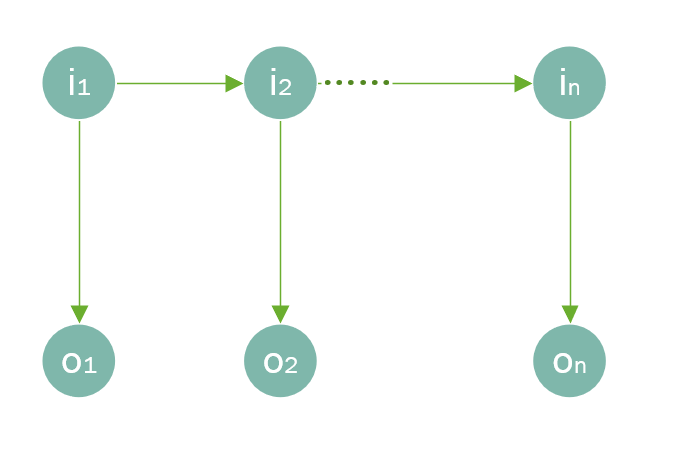二、隐马尔科夫模型原理

HHM就是基于这种马尔科夫链的，即基于以上的假设，所以它的联合概率分布为：

P ( o 1 , i 1 , . . . , o T , i T ) = P ( i 1 ) P ( o 1 ∣ i 1 ) ∏ i = 2 T P ( i i ∣ i i − 1 ) P ( o i ∣ i i ) P(o_1,i_1,...,o_T,i_T) = P(i_1)P(o_1|i_1)\prod_{i=2}^T P(i_i|i_{i-1})P(o_i|i_i)

这就是HHM的模型结构了。一个模型肯定还包含参数，机器学习的本质就是找到一组最优的参数，使得模型的拟合效果最好，HHM也不例外。

那么，HHM的参数包括以下3组：

1. 状态转移概率A： a i j = P ( i t + 1 = q j ∣ i t = q i ) , 1 < = i , j < = N a_{ij} = P(i_{t+1}=q_j|i_t=q_i), 1<=i,j<=N ，即t时刻状态为 q i q_i 时，t+1时刻状态为 q j q_j 的概率
2. 输出观测概率B： b j ( k ) = P ( o t = q k ∣ i t = q j ) , 1 < = j < = N , 1 < = k < = M b_j(k) = P(o_t=q_k|i_t=q_j), 1<=j<=N,1<=k<=M ，即t时刻的状态为 q i q_i 时，观测值为 o j o_j 的概率
3. 初设状态概率 π i \pi_i π = ( π 1 , π 2 , . . . , π N ) , 1 < = i < = N \pi = (\pi_1, \pi_2,...,\pi_N), 1<=i<=N ，即初设时刻各状态出现的概率。

那么，给定一个隐马尔科夫模型，它产生观测序列的步骤如下 { o 1 , o 2 , . . . . , o T } \{o_1, o_2,....,o_T\}

(1) 当t = 1时，根据初设状态概率 π \pi 选择初设状态 i 1 i_1

(2) 根据状态 i t i_t 和输出观测概率B选择观测值 o t o_t

(3) 根据状态 i t i_t 和状态转移概率A确定 i t + 1 i_{t+1}

(4) 当t < T，令t = t + 1，并跳转至第2步，否则终止，观测序列已全部生产完毕。

三个基本问题

1. 概率计算问题。给定模型 λ = ( A , B , π ) \lambda=(A,B,\pi) 和观测序列 O = { o 1 , o 2 , . . . . , o T } O = \{o_1, o_2,....,o_T\} ，计算观测序列O出现的概率 P ( O ∣ λ ) P(O|\lambda)
2. 学习问题。已知观测序列 O = { o 1 , o 2 , . . . . , o T } O = \{o_1, o_2,....,o_T\} ，估计模型参数 λ = ( A , B , π ) \lambda=(A,B,\pi) ，使得观测序列概率 P ( O ∣ λ ) P(O|\lambda) 最大
3. 预测问题。给定模型 λ = ( A , B , π ) \lambda=(A,B,\pi) 和观测序列 O = { o 1 , o 2 , . . . . , o T } O = \{o_1, o_2,....,o_T\} ，求对给定观测序列条件概率P(I|O)最大的隐藏状态 I = { i 1 , i 2 , . . . . . , i T } I = \{i_1, i_2,....., i_T\}

三、概率计算

1. 前向算法

给定隐马尔科夫模型 λ \lambda ，至时刻t的观测序列为 { o 1 , o 2 , . . . . , o t } \{o_1, o_2,....,o_t\} ，且状态为 q i q_i 的概率为前向概率：

α t ( i ) = P ( o 1 , o 2 , . . . . , o t , i t = q i ∣ λ ) \alpha_t(i)=P(o_1, o_2,....,o_t,i_t=q_i|\lambda)

我们可以通过递推的方式求得前向概率 α t ( i ) \alpha_t(i) 及观测序列概率 P ( O ∣ λ ) P(O|\lambda) ，具体的算法步骤如下：

(1) 时刻t=1， α 1 ( i ) = π i b i ( o 1 ) , i = 1 , 2 , . . . , N \alpha_1(i)=\pi_ib_i(o_1),i=1,2,...,N

(2) 对于时刻 t = 1 , 2 , . . . , T − 1 t=1,2,...,T-1

α t + 1 ( i ) = [ ∑ j = 1 N α t ( j ) a j i ] b i ( o t + 1 ) , i = 1 , 2 , . . . , N \alpha_{t+1}(i)=\left[\sum_{j=1}^N\alpha_t(j)a_{ji}\right]b_i(o_{t+1}),i=1,2,...,N

(3) P ( O ∣ λ ) = ∑ i = 1 N α T ( i ) P(O|\lambda)=\sum^N_{i=1}\alpha_T(i)

2. 后向算法

给定隐马尔科夫模型 λ \lambda ，满足时刻t的状态为 q i q_i 的条件下，从时刻t+1到T的观测序列为 { o t + 1 , o t + 2 , . . . . , o T } \{o_{t+1}, o_{t+2},....,o_T\} 的概率为后向概率：

β t ( i ) = P ( o t + 1 , o t + 2 , . . . . , o T ∣ i t = q i , λ ) \beta_t(i)=P(o_{t+1}, o_{t+2},....,o_T|i_t=q_i,\lambda)

我们同样可以通过递推的方式求得前向概率 β t ( i ) \beta_t(i) 及观测序列概率 P ( O ∣ λ ) P(O|\lambda) ，具体的算法步骤如下：

(1) 对于最后时刻T，规定 β T ( i ) = 1 \beta_T(i)=1

(2) 对于时刻t=T-1, T-2, … , 1：

β t ( i ) = ∑ j = 1 N a i j b j ( o t + 1 ) β t + 1 ( j ) , i = 1 , 2 , . . . , N \beta_t(i)=\sum^N_{j=1}a_{ij}b_j(o_{t+1})\beta_{t+1}(j), i=1,2,...,N

(3) P ( O ∣ λ ) = ∑ i = 1 N π i b i ( o 1 ) β 1 ( i ) P(O|\lambda)=\sum^N_{i=1}\pi_ib_i(o_1)\beta_1(i)

这里我们可以这里理解 ： β 1 ( i ) \beta_1(i) 表示时刻t=1的状态为 q 1 q_1 时，从时刻t=2到T的观测序列为 { o 2 , o 3 , . . . . , o T } \{o_{2}, o_{3},....,o_T\} 的概率， π i b i ( o 1 ) \pi_ib_i(o_1) 当然就是时刻t=1的状态为 q 1 q_1 的概率了，那么 π i b i ( o 1 ) β 1 ( i ) \pi_ib_i(o_1)\beta_1(i) 也就是时刻t=1的状态为 q 1 q_1 的条件下的条件概率了。

但是观测序列概率是没有限定观测序列的状态取值的，所以，要把所有状态下的观测序列概率累加起来才是最后的观测序列概率，即上式。

这里跟上面的前向算法求得的观测序列概率的思想是类似的。

3. 常用的概率计算

1. 根据已知的隐马尔科夫模型 λ \lambda 和观测序列O，在时刻 t 处于状态 q i q_i 的概率：

γ t ( i ) = P ( i t = q i ∣ O , λ ) \gamma_t(i)=P(i_t=q_i|O,\lambda)

并且，根据条件概率的公式进行推导可知：

γ t ( i ) = P ( i t = q i ∣ O , λ ) = P ( i t = q i , O ∣ λ ) P ( O ∣ λ ) \gamma_t(i)=P(i_t=q_i|O,\lambda)=\frac{P(i_t=q_i,O|\lambda)}{P(O|\lambda)}

再结合前向和后向概率，可得到：

P ( i t = q i , O ∣ λ ) = α t ( i ) β t ( i ) P(i_t=q_i,O|\lambda)=\alpha_t(i)\beta_t(i)

（这里多琢磨几遍前向概率和后向概率的定义，就可以理解为什么了）

则：

γ t ( i ) = α t ( i ) β t ( i ) P ( O ∣ λ ) = α t ( i ) β t ( i ) ∑ j = 1 N α t ( j ) β t ( j ) \gamma_t(i)=\frac{\alpha_t(i)\beta_t(i)}{P(O|\lambda)}=\frac{\alpha_t(i)\beta_t(i)}{\sum^N_{j=1}\alpha_t(j)\beta_t(j)}

（分母的转换是这么理解：在已知 λ \lambda 的情况下，观测序列为O时，状态可以为任何一种状态，那么每一种状态下观测序列为O的概率累加，就是观测序列的概率了）

2. 根据已知的隐马尔科夫模型 λ \lambda 和观测序列O，在时刻 t 处于状态 q i q_i ，并且在时刻t+1处于状态 q j q_j 的概率：

ξ t ( i , j ) = P ( i t = q i , i t + 1 = q j ∣ O , λ ) \xi_t(i,j)=P(i_t=q_i,i_{t+1}=q_j|O,\lambda)

跟上面的思路一致：

ξ t ( i , j ) = P ( i t = q i , i t + 1 = q j , O ∣ λ ) P ( O ∣ λ ) = P ( i t = q i , i t + 1 = q j , O ∣ λ ) ∑ i = 1 N ∑ j = 1 N P ( i t = q i , i t + 1 = q j , O ∣ λ ) \xi_t(i,j)=\frac{P(i_t=q_i,i_{t+1}=q_j,O|\lambda)}{P(O|\lambda)}=\frac{P(i_t=q_i,i_{t+1}=q_j,O|\lambda)}{\sum^N_{i=1}\sum^N_{j=1}P(i_t=q_i,i_{t+1}=q_j,O|\lambda)}

P ( i t = q i , i t + 1 = q j , O ∣ λ ) = α t ( i ) a i j b j ( o t + 1 ) β t + 1 ( j ) P(i_t=q_i,i_{t+1}=q_j,O|\lambda)=\alpha_t(i)a_{ij}b_j(o_{t+1})\beta_{t+1}(j)

三、实际应用

可能看了上面隐马尔科夫模型的原理之后，很多人都有点懵，不知道能用来做什么？

我们举个例子：中文分词。

“我们是中国人”，这一句话分词之后应该是这样的：我(B)们(E)|是(O)|中(B)国(M)人(E)。

各个标签的含义——B：单词的第一个字，M：单词的中间字，E：单词的最后一个字，O：仅有一个字组成的词。

将隐马尔科夫模型应用到分词中，那么观测序列对应的是文本句子，隐藏状态对应则的是句子中每个字的标签。

四、模型训练(学习)

上面我们提到了，HHM的参数有三组 A , B , π {A,B,\pi} ，那么如何确定最优的参数呢？

1. 监督学习

当我们的样本数据是带有标签时，即训练数据包含观测序列以及对应的状态，我们就可以通过极大似然法来估计HHM的参数，计算方法如下：

(1) 状态转移概率A: a ^ i j = A i j ∑ k = 1 N A i k , 1 < = i , j < = N \hat{a}_{ij} = \frac{A_{ij}}{\sum_{k=1}^NA_{ik}},1<=i,j<=N

A i j A_{ij} 表示样本中时刻t处于状态 q i q_i 转移到时刻t+1处于状态 q j q_j 的频次。

如上面分词的例子， A i j A_{ij} 可以理解为当前这个字的标签为 q i q_i (假如为B)，下一个词的标签为 q j q_j （假如为E）的频次。

(2) 输出观测概率B： b ^ i j = B i j ∑ k = 1 N B i k , 1 < = i < = N , 1 < = j < = M \hat{b}_{ij} = \frac{B_{ij}}{\sum_{k=1}^NB_{ik}},1<=i<=N,1<=j<=M

B i j B_{ij} 表示样本中t时刻的状态为 q i q_i 时，观测值为 o j o_j 的频次。

如当前的字为 o j o_j （假如为"我"），标签为 q i q_i （假如为B）的频次。

(3) 初设状态概率 π \pi π ^ i \hat{\pi}_i 为初设状态为 q i q_i 的频率。

2. Baum-Welch算法

如果样本数据时没有带标签的，即训练数据只包含观测序列O，但对应的状态 I I 未知。

那此时的隐马尔科夫模型是一个含有隐变量的概率模型：

P ( O ∣ λ ) = ∑ I P ( O ∣ I , λ ) P ( I ∣ λ ) P(O|\lambda)=\sum_IP(O|I,\lambda)P(I|\lambda)

它的参数学习可以由Baum-Welch算法实现，本质还是EM。EM的基本思想是先将参数的初设估计值加入到似然函数Q中，然后对Q进行极大化（一般就是求导，令其等于0），得到新的参数估计值，一直重复，直到收敛。

此时，对数似然函数是 l o g P ( O , I ∣ λ ) logP(O,I|\lambda)

(1) EM算法的E步：求Q函数：

Q ( λ , λ ˉ ) = ∑ I l o g P ( O , I ∣ λ ) P ( O , I ∣ λ ˉ ) Q(\lambda,\bar{\lambda})=\sum_IlogP(O,I|\lambda)P(O,I|\bar{\lambda})

λ \lambda 是要极大化的隐马尔科夫模型的参数， λ ˉ \bar{\lambda} 是参数的当前估计值。

因为 P ( O , I ∣ λ ) = π i 1 b i 1 ( o 1 ) a i 1 i 2 b i 2 . . . a i T − 1 i T b i T ( o T ) P(O,I|\lambda)=\pi_{i1}b_{i_1}(o_1)a_{i_1i_2}b_{i_2}...a_{i_{T-1}i_T}b_{i_T}(o_T)

因此 Q ( λ , λ ˉ ) = ∑ I l o g π i 1 P ( O , I ∣ λ ˉ ) + ∑ I ( ∑ t = 1 T − 1 l o g α i t i t + 1 ) P ( O , I ∣ λ ˉ ) + ∑ I ( ∑ t = 1 T l o g b i t ( o t ) ) P ( O , I ∣ λ ˉ ) Q(\lambda,\bar{\lambda})=\sum_Ilog\pi_{i_1}P(O,I|\bar{\lambda})+\sum_I\left(\sum^{T-1}_{t=1}log\alpha_{i_ti_{t+1}}\right)P(O,I|\bar{\lambda})+\sum_I\left(\sum^T_{t=1}logb_{i_t}(o_t)\right)P(O,I|\bar{\lambda})

(2) EM算法的M步：极大化Q函数，得到新的参数估计值 A , B , λ A,B,\lambda

由于三个参数单独出现在Q函数的3个项中，那么我们可以对各项分别极大化。

a. 首先是第1项求 λ \lambda

∑ I l o g π i 1 P ( O , I ∣ λ ˉ ) = ∑ i = 1 N l o g π i P ( O , i 1 = i ∣ λ ˉ ) \sum_Ilog\pi_{i_1}P(O,I|\bar{\lambda})=\sum^N_{i=1}log\pi_iP(O,i_1=i|\bar{\lambda})

为什么这个等式成立呢？因为当隐马尔科夫模型的参数确定后，时刻t=1的状态 i 1 i_1 确定了，那么O和 I I 都能确定，这里是把 i 1 i_1 的所有独立事件概率加起来

π i \pi_i 满足约束条件 ∑ i = 1 N π = 1 \sum^N_{i=1}\pi=1 ，可以利用拉格朗日乘子法，拉格朗日函数为：

∑ i = 1 N l o g π i P ( O , i 1 = i ∣ λ ˉ ) + γ ( ∑ i = 1 N π i − 1 ) \sum^N_{i=1}log\pi_iP(O,i_1=i|\bar{\lambda})+\gamma\left(\sum^N_{i=1}\pi_i-1\right)

求偏导并令其等于0：

∂ ∂ π i [ ∑ i = 1 N l o g π i P ( O , i 1 = i ∣ λ ˉ ) + γ ( ∑ i = 1 N π i − 1 ) ] = 0 \frac{\partial}{\partial \pi_i}\left[\sum^N_{i=1}log\pi_iP(O,i_1=i|\bar{\lambda})+\gamma\left(\sum^N_{i=1}\pi_i-1\right)\right]=0

得到： P ( O , i 1 = i ∣ λ ˉ ) + γ π i = 0 P(O,i_1=i|\bar{\lambda})+\gamma\pi_i=0

对所有i求和，得到： γ = − P ( O ∣ λ ˉ ) \gamma=-P(O|\bar{\lambda})

因为 i 1 ∈ ( 1 , 2 , . . . , N ) i_1\in(1,2,...,N) ，所以 P ( O , i 1 = i ∣ λ ˉ ) P(O,i_1=i|\bar{\lambda}) 中按 i i 从1到N累计起来其实就等于 P ( O ∣ λ ˉ ) P(O|\bar{\lambda})

最终， π i = P ( O , i 1 = i ∣ λ ˉ ) P ( O ∣ λ ˉ ) \pi_i=\frac{P(O,i_1=i|\bar{\lambda})}{P(O|\bar{\lambda})}

b. Q函数的第2项求a：

∑ I ( ∑ t = 1 T − 1 l o g α i t i t + 1 ) P ( O , I ∣ λ ˉ ) = ∑ i = 1 N ∑ j = 1 N ∑ t = 1 T − 1 a i j P ( O , i t = i , i t + 1 = j ∣ λ ˉ ) \sum_I\left(\sum^{T-1}_{t=1}log\alpha_{i_ti_{t+1}}\right)P(O,I|\bar{\lambda})=\sum^N_{i=1}\sum^N_{j=1}\sum^{T-1}_{t=1}a_{ij}P(O,i_t=i,i_{t+1}=j|\bar{\lambda})

同样存在约束条件： ∑ j = 1 N a i j = 1 \sum^N_{j=1}a_{ij}=1 ，类似上面的做法，可以求得：

a i j = ∑ t = 1 T − 1 P ( O , i t = i , i t + 1 = j ∣ λ ˉ ) ∑ t = 1 T − 1 P ( O , i t = i ) ∣ λ ˉ a_{ij}=\frac{\sum^{T-1}_{t=1}P(O,i_t=i,i_{t+1}=j|\bar{\lambda})}{\sum^{T-1}_{t=1}P(O,i_t=i)|\bar{\lambda}}

c. Q函数的第3项求b：

∑ I ( ∑ t = 1 T l o g b i t ( o t ) ) P ( O , I ∣ λ ˉ ) = ∑ j = 1 N ∑ t = 1 T l o g b j ( o t ) i P ( O , i t = j ∣ λ ˉ ) \sum_I\left(\sum^T_{t=1}logb_{i_t}(o_t)\right)P(O,I|\bar{\lambda})=\sum^N_{j=1}\sum^T_{t=1}logb_j(o_t)_iP(O,i_t=j|\bar{\lambda})

约束条件： ∑ k = 1 M b j ( k ) = 1 \sum^M_{k=1}b_j(k)=1 ，这里需要注意只有当 o t = v k o_t=v_k b j ( o t ) b_j(o_t) b j ( k ) b_j(k) 的偏导数才不等于0，用 I ( o t = v k ) I(o_t=v_k) 表示，求得：

b j ( k ) = ∑ t = 1 T P ( O , i t = j ∣ λ ˉ ) I ( o t = v k ) ∑ t = 1 T P ( O , i t = j ∣ λ ˉ ) b_j(k)=\frac{\sum^T_{t=1}P(O,i_t=j|\bar{\lambda})I(o_t=v_k)}{\sum^T_{t=1}P(O,i_t=j|\bar{\lambda})}

(3) 最后，用上面的概率表示：

a i j = ∑ t = 1 T − 1 ξ t ( i , j ) ∑ t = 1 T − 1 γ t ( i ) a_{ij}=\frac{\sum^{T-1}_{t=1}\xi_t(i,j)}{\sum^{T-1}_{t=1}\gamma_t(i)}

b j ( k ) = ∑ t = 1 , o t = v k T γ t ( j ) ∑ t = 1 T γ t ( j ) b_j(k)=\frac{\sum^T_{t=1,o_t=v_k}\gamma_t(j)}{\sum^T_{t=1}\gamma_t(j)}

π i = γ 1 ( i ) \pi_i=\gamma_1(i)

五、模型预测

那么，通过训练，我们得到了最优的参数，也相当于我们得到了一个拟合效果最好的隐马尔科夫模型，此时我们如何预测观测序列的状态呢？即给定隐马尔科夫模型 λ = [ A , B , π ] \lambda=[A,B,\pi] ，如何通过观测序列来推断对应的隐藏状态。

举个例子：例如我们这个模型用于语音识别中，那么观测序列就是语音了，隐藏状态即为文字。语音识别的作用就是将语音转化为对应的文字，那如果隐马尔科夫模型通过观测序列得到隐藏状态，不就是将语音转化为文字了。

这个时候就需要使用到维特比(Viterbi)算法，在这篇博客中有详细介绍。但在隐马尔科夫模型中需要稍作调整，下面我给出书上的一个例子。

维特比(Viterbi)算法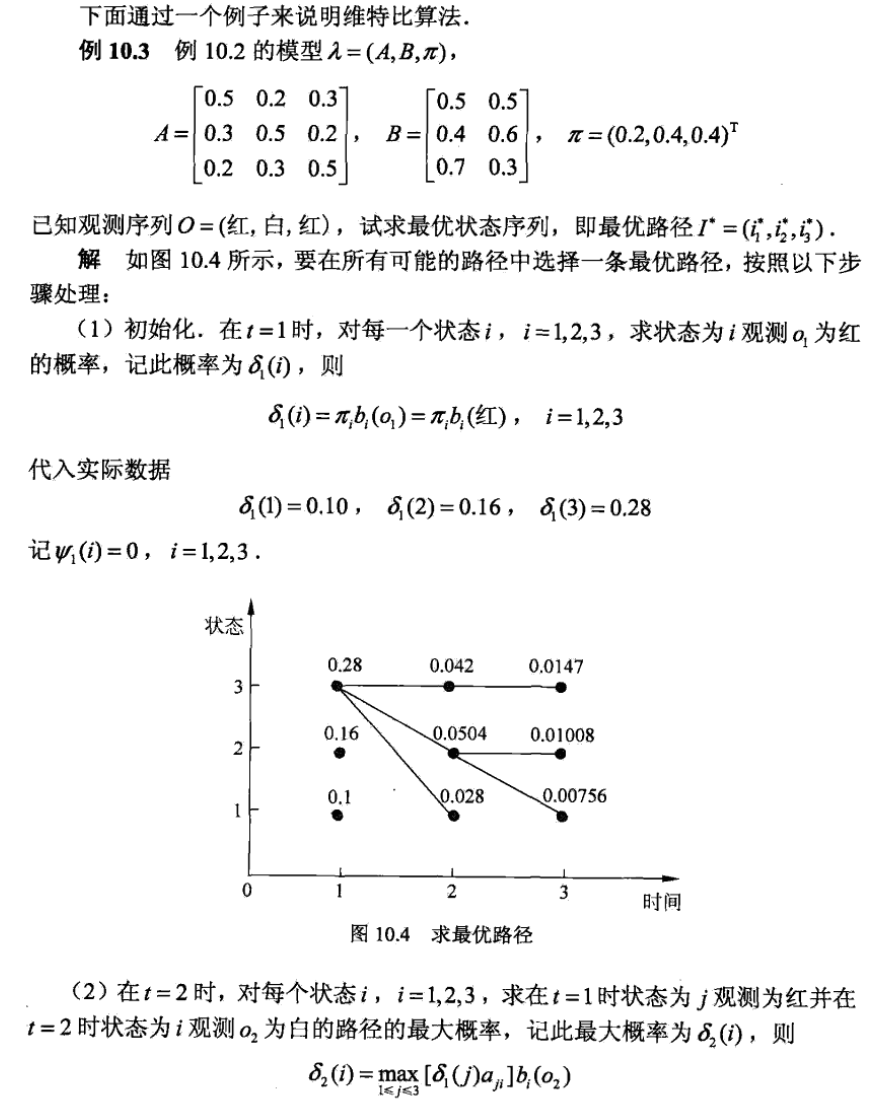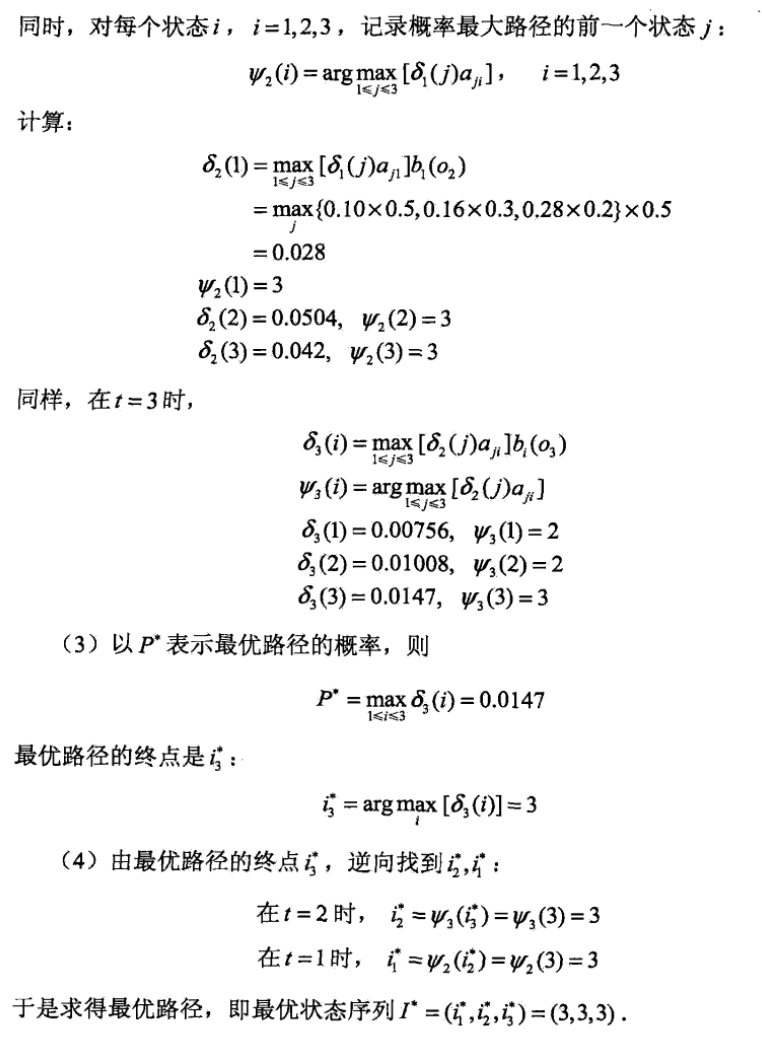欢迎关注同名公众号：“我就算饿死也不做程序员”。
交个朋友，一起交流，一起学习，一起进步。展开全文sgyuanshi 2020-04-29 21:04:10
• 89KB weixin_42696333 2021-09-10 17:33:12
• MATLAB编写的学习隐马尔科夫模型的程序 matlab

32KB qq_43177596 2018-09-11 09:19:38
• 593KB weixin_38664556 2020-02-09 14:53:36
• 通俗理解什么是隐马尔科夫模型(hmm) 隐马尔科夫模型 机器学习 自然语言处理 分词

文章目录什么是隐马尔科夫模型HMM模型相关的算法前向算法(forward algorithm)维特比算法(Viterbi algorithm)求解最可能的天气NLP应用小结 参考文章一文搞懂HMM（隐马尔可夫模型） 本人博客地址: ...

参考文章 一文搞懂HMM（隐马尔可夫模型）
本人博客地址: https://xiaoxiablogs.top

什么是隐马尔科夫模型

隐马尔可夫模型（Hidden Markov Model，HMM）是统计模型，它用来描述一个含有隐含未知参数的马尔可夫过程。其难点是从可观察的参数中确定该过程的隐含参数。然后利用这些参数来作进一步的分析，例如模式识别。用一个简单的例子来描述:

假设有3个不同的骰子，分别为四面(D4)、六面(D6)、八面(D8)，每个面的概率都是相同的，每个骰子被选中的概率也是相同的。

假设我们开始掷骰子，随机从三个里面选一个，然后掷骰子，我们会得到一个数字，重复上面的过程，我们就可以得到一串数字。例如我们得到的是1 8 6 4 4 2 3 6 1 4，这串数字就是可见状态链，而我们对应的掷骰子的顺序就是隐含状态链，假设为D4 D8 D6 D4 D6 D8 D6 D6 D4 D8，我们第一次随机的骰子是D4, 而第一次选中每个骰子的概率是1/3，也就是初始概率， 而第一次选中D4后，第二次选中每个骰子的概率为1/3，这就是转换概率，如:D4->D8为1/3，即选中D4后再选中D8的转移概率为1/3。

一般来说，HMM中说到的马尔科夫链其实就是指隐含状态链，因为隐含状态之间存在转换概率

HMM模型相关的算法

1. 知道隐含状态数量、转换概率、可见状态链、输出概率，求隐含状态链
2. 知道隐含状态数量、转换概率、可见状态链、输出概率，求这个结果的概率
3. 知道隐含状态数量、隐含状态链、可见状态链、输出概率，求转换概率

前向算法(forward algorithm)

我们拿到一串可见状态链，也知道转换概率、输出概率，如何求解隐含状态链最可能是什么呢?

我们就可以使用前向算法(forward algorithm)

例如我们得到的数字为: 1 6 3

我们可以分为以下几步:

首先，我们只掷一次骰子:看到结果为1，产生这个结果的总概率可以按照如下计算，清高率为0.18：然后我们掷两次骰子:看到结果为1，6.产生这个结果的总概率可以按照如下计算，总概率为0.05：然后我们掷三次骰子:看到结果为1，6，3.产生这个结果的总概率可以按照如下计算，总概率为0.03：同样的，我们一步一步的算，有多长算多长，再长的马尔可夫链总能算出来的。这就是前向算法(forward algorithm)

维特比算法(Viterbi algorithm)

任何一个HMM都可以通过下列五元组来描述：

:param obs:观测序列
:param states:隐状态
:param start_p:初始概率（隐状态）
:param trans_p:转移概率（隐状态）
:param emit_p: 发射概率 （隐状态表现为显状态的概率）states = ('Rainy', 'Sunny')

observations = ('walk', 'shop', 'clean')

start_probability = {'Rainy': 0.6, 'Sunny': 0.4}

transition_probability = {
'Rainy' : {'Rainy': 0.7, 'Sunny': 0.3},
'Sunny' : {'Rainy': 0.4, 'Sunny': 0.6},
}

emission_probability = {
'Rainy' : {'walk': 0.1, 'shop': 0.4, 'clean': 0.5},
'Sunny' : {'walk': 0.6, 'shop': 0.3, 'clean': 0.1},
}

求解最可能的天气

求解最可能的隐状态序列是HMM的三个典型问题之一，通常用维特比算法解决。维特比算法就是求解HMM上的最短路径（-log(prob)，也即是最大概率）的算法。

稍微用中文讲讲思路，很明显，第一天天晴还是下雨可以算出来：

1. 定义V[时间][今天天气] = 概率，注意今天天气指的是，前几天的天气都确定下来了（概率最大）今天天气是X的概率，这里的概率就是一个累乘的概率了。
2. 因为第一天我的朋友去散步了，所以第一天下雨的概率V[第一天][下雨] = 初始概率[下雨] * 发射概率[下雨][散步] = 0.6 * 0.1 = 0.06，同理可得V[第一天][天晴] = 0.24 。从直觉上来看，因为第一天朋友出门了，她一般喜欢在天晴的时候散步，所以第一天天晴的概率比较大，数字与直觉统一了。
3. 从第二天开始，对于每种天气Y，都有前一天天气是X的概率 * X转移到Y的概率 * Y天气下朋友进行这天这种活动的概率。因为前一天天气X有两种可能，所以Y的概率有两个，选取其中较大一个作为V[第二天][天气Y]的概率，同时将今天的天气加入到结果序列中
4. 比较V[最后一天][下雨]和[最后一天][天晴]的概率，找出较大的哪一个对应的序列，就是最终结果。
# -*- coding:utf-8 -*-
# Filename: viterbi.py
# Author：hankcs
# Date: 2014-05-13 下午8:51

states = ('Rainy', 'Sunny')

observations = ('walk', 'shop', 'clean')

start_probability = {'Rainy': 0.6, 'Sunny': 0.4}

transition_probability = {
'Rainy' : {'Rainy': 0.7, 'Sunny': 0.3},
'Sunny' : {'Rainy': 0.4, 'Sunny': 0.6},
}

emission_probability = {
'Rainy' : {'walk': 0.1, 'shop': 0.4, 'clean': 0.5},
'Sunny' : {'walk': 0.6, 'shop': 0.3, 'clean': 0.1},
}

# 打印路径概率表
def print_dptable(V):
print "    ",
for i in range(len(V)): print "%7d" % i,
print

for y in V.keys():
print "%.5s: " % y,
for t in range(len(V)):
print "%.7s" % ("%f" % V[t][y]),
print

def viterbi(obs, states, start_p, trans_p, emit_p):
"""

:param obs:观测序列
:param states:隐状态
:param start_p:初始概率（隐状态）
:param trans_p:转移概率（隐状态）
:param emit_p: 发射概率 （隐状态表现为显状态的概率）
:return:
"""
# 路径概率表 V[时间][隐状态] = 概率
V = [{}]
# 一个中间变量，代表当前状态是哪个隐状态
path = {}

# 初始化初始状态 (t == 0)
for y in states:
V[y] = start_p[y] * emit_p[y][obs]
path[y] = [y]

# 对 t > 0 跑一遍维特比算法
for t in range(1, len(obs)):
V.append({})
newpath = {}

for y in states:
# 概率 隐状态 =    前状态是y0的概率 * y0转移到y的概率 * y表现为当前状态的概率
(prob, state) = max([(V[t - 1][y0] * trans_p[y0][y] * emit_p[y][obs[t]], y0) for y0 in states])
# 记录最大概率
V[t][y] = prob
# 记录路径
newpath[y] = path[state] + [y]

# 不需要保留旧路径
path = newpath

print_dptable(V)
(prob, state) = max([(V[len(obs) - 1][y], y) for y in states])
return (prob, path[state])

def example():
return viterbi(observations,
states,
start_probability,
transition_probability,
emission_probability)

print example()

输出:

0       1       2
Rainy:  0.06000 0.03840 0.01344
Sunny:  0.24000 0.04320 0.00259
(0.01344, ['Sunny', 'Rainy', 'Rainy'])

NLP应用

具体到分词系统，可以将天气当成“标签”，活动当成“字或词”。那么，几个NLP的问题就可以转化为：

• 词性标注：给定一个词的序列（也就是句子），找出最可能的词性序列（标签是词性）。如ansj分词和ICTCLAS分词等。
• 分词：给定一个字的序列，找出最可能的标签序列（断句符号：[词尾]或[非词尾]构成的序列）。结巴分词目前就是利用BMES标签来分词的，B（开头）,M（中间),E(结尾),S(独立成词）
• 命名实体识别：给定一个词的序列，找出最可能的标签序列（内外符号：[内]表示词属于命名实体，[外]表示不属于）。如ICTCLAS实现的人名识别、翻译人名识别、地名识别都是用同一个Tagger实现的。

小结

HMM是一个通用的方法，可以解决贴标签的一系列问题。

展开全文c2861024198 2021-06-15 15:41:19
• 马尔科夫链代码，关于matlab隐马尔科夫模型工具箱的 matlab

41KB qq_43177596 2018-09-11 09:11:48
• 967KB yumenzhonga 2014-08-10 21:26:47
• 附件为HMM隐马尔科夫模型——学习问题 机器学习

3KB lfc690585553 2018-08-09 15:26:01
• HMM隐马尔科夫模型及股票预测 深度学习 马尔科夫链 股票预测 HMM

隐马尔科夫模型是自然语言处理中很重要的一种算法。在此之前，我们首先给大家介绍马尔科夫链。马尔科夫链，因安德烈·马尔科夫得名，是指数学中具有马尔科夫性质的离散事件的随机过程。在给定当前知识或信息的情况下...

0 前言

不少同学让我出一起HMM的教学文章，学长为了满足各位同学的要求，写下此文

毕设帮助，开题指导，技术解答
🇶746876041

1 马尔科夫链

隐马尔科夫模型是自然语言处理中很重要的一种算法。在此之前，我们首先给大家介绍马尔科夫链。马尔科夫链，因安德烈·马尔科夫得名，是指数学中具有马尔科夫性质的离散事件的随机过程。在给定当前知识或信息的情况下，过去对于预测未来是无关的。每个状态的转移只依赖于之前的N个状态，这个过程被称为1个n阶的模型。其中n是影响转移状态的数目。最简单的马尔科夫过程就是一阶过程，每一个状态的转移只依赖于其之前的哪一个状态。用数学的表达式表示就是如下所示：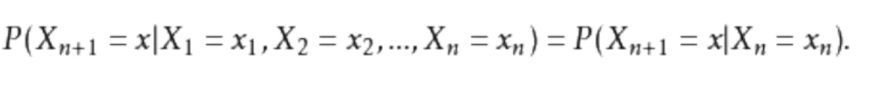假设天气服从马尔科夫链：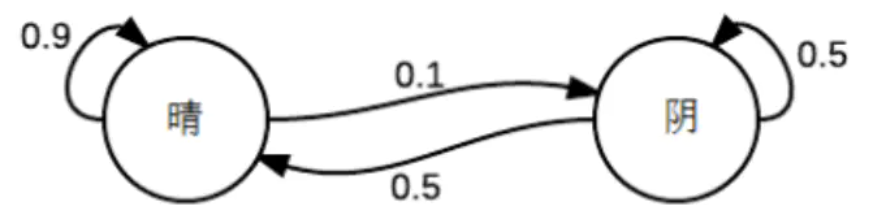转移矩阵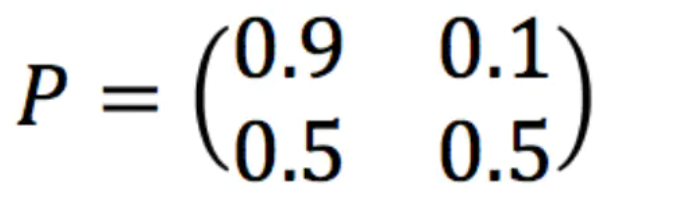那么，从今天开始，在遥远的未来的某天，晴阴的概率分布是什么？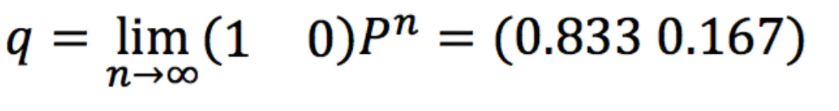其实，不管是今天是晴天，还是阴天，其实很多天之后的晴阴分布收敛到一个固定分布。这个固定分布就是我们熟悉的稳态分布。因此，我们就为上面的一阶马尔科夫过程定义一以下三个部分：

• 1、状态：晴天、阴天
• 2、初始向量：定义系统在时间为0的时候的状态概率
• 3、状态转移矩阵：每种天气转换的概率。所有的能被这样描述的系统就是一个马尔科夫过程。

但是

以上出现一个明显的缺陷，也就是前后关系的缺失，带来了信息的缺失：例如我们经常玩的股市，如果只是观测市场，我们只能知道当天的价格，成交量等信息，但是并不知道当前的股市是处于什么状态。

在这种情况下我们有两个状态集合，一个是可以观察到的状态集合；另一个是一个隐藏的状态集合。我们希望能够找到一个算法可以根据股市价格的成交量状况和马尔科夫假设来预测股市的状况。基于上面我们提到的情况下，我们可以观察到状态序列和隐藏的状态序列是概率相关的。因此，我们可以将这种类型的过程建模为一个有隐藏的马尔科夫过程和一个与这个隐马尔科夫过程概率相关的并且可以观察到的状态集合，这就是我们今天要介绍的隐马尔科夫模型(HMM)。

接下学长来为大家详细介绍隐马尔科夫模型。

2 隐马尔科夫模型

隐马尔科夫模型是一种统计模型，用来描述一个含有隐含层未知参数的马尔科夫过程。但是其中最大的难点就是从可观察的参数中确定该过程的隐含参数，然后利用这些参数来作进一步分析。我们以日常的投骰子为例来解释隐马尔科夫模型：假设有三个不同的骰子(6面、4面、8面)，每次先从三个骰子里面选择一个，每个骰子选中的概率为1/3，如下图所示，重复上述过程，得到一串数值[1,6,3,5,2,7]。这些可观测变量组成可观测状态链。同时，在隐马尔可夫模型中还有一条由隐变量组成的隐含状态链，在本例中即骰子的序列。比如得到这串数字骰子的序列可能为[D6, D8, D8, D6, D4, D8]。

具体如下：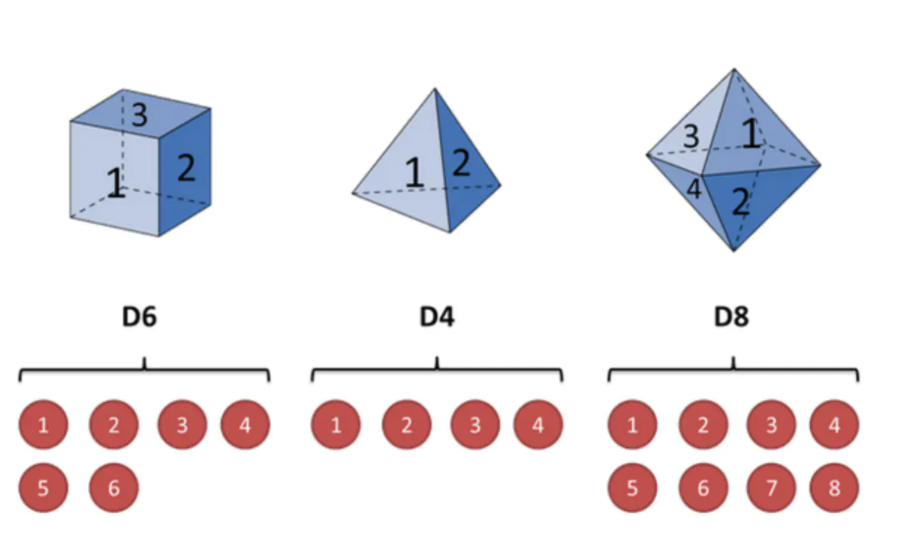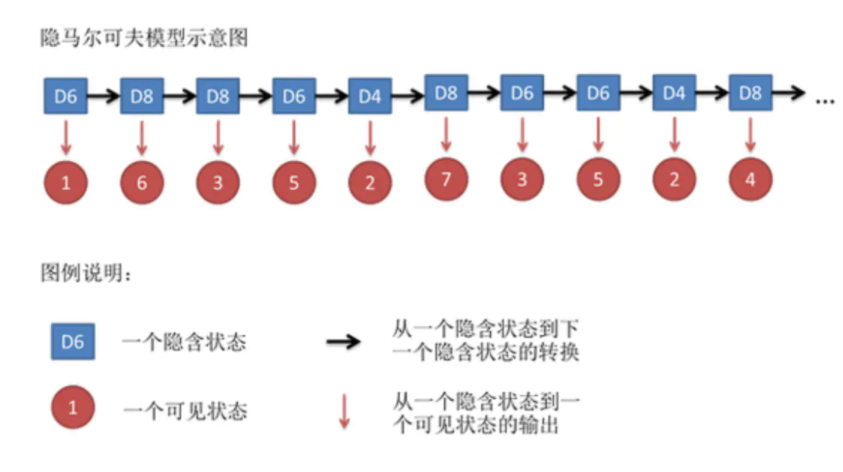隐含状态转换示意图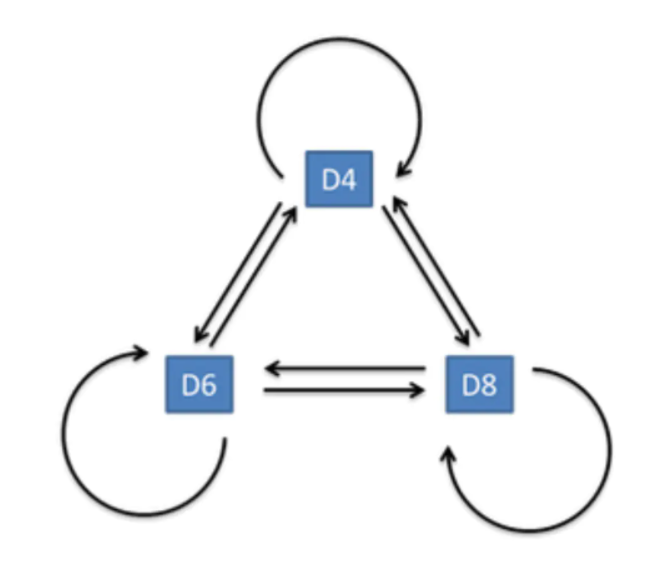3 HMM预测股票

这次使用HMM算法来进行股票预测，使用昨天的股票数据来预测今天是否涨跌。

import tushare as ts

token = ''  # 设置tocken
pro = ts.pro_api(token)  # 初始化pro接口
# 获取数据
df_gldq = pro.daily(ts_code='000651.sz', start_date='20201210', end_date='20210513')

# 打印数据
df_gldq.to_excel('data/stock.xls')

首先使用tushare模块来获取数据。token是自己获取的，ts_code决定哪支股票，还有时间区间以及排序，最后写入stock.xls文件中。

#数据处理
print("原始数据的大小：", df.shape)
print("原始数据的列名", df.columns)

df.reset_index(inplace=True, drop=False)
df.drop(['index', 'ts_code', 'open', 'high', 'low' , 'pre_close', 'change', 'pct_chg', 'amount'], axis=1, inplace=True)
close_v = df['close']
volume = df['vol'][1:]
diff = np.diff(close_v)
#获得输入数据
X = np.column_stack([diff, volume])
print("输入数据的大小：", X.shape)   #(1504, 2)

min = X.mean(axis=0) - 8*X.std(axis=0)   #最小值
max = X.mean(axis=0) + 8*X.std(axis=0)  #最大值
X = pd.DataFrame(X)
#异常值设为均值
for i in range(len(X)):  #dataframe的遍历
if (X.loc[i, 0]< min) | (X.loc[i, 0] > max):
X.loc[i, 0] = X.mean(axis=0)

#数据集的划分
X_Test = X.iloc[:-30]
X_Pre = X.iloc[-30:]
print("训练集的大小：", X_Test.shape)     #(1474, 2)
print("测试集的大小：", X_Pre.shape)      #(30, 2)

接下来是数据的预处理。获取特征因子与标签，归一化、异常值处理、数据集划分等等。

model = GaussianHMM(n_components=8, covariance_type='diag', n_iter=1000, min_covar=0.1)
model.fit(X_Test)

expected_returns_volumes = np.dot(model.transmat_, model.means_)
expected_returns = expected_returns_volumes[:,0]
predicted_price = []  #预测值
current_price = close_v.iloc[-30]
for i in range(len(X_Pre)):
hidden_states = model.predict(X_Pre.iloc[i].values.reshape(1,2))  #将预测的第一组作为初始值
predicted_price.append(current_price+expected_returns[hidden_states])
current_price = predicted_price[i]

然后是利用HMM做股票预测

x = dates[-29: ]
y_act = close_v[-29:]
y_pre = pd.Series(predicted_price[:-1])
plt.figure(figsize=(8,6))
plt.plot_date(x, y_act,linestyle="-",marker="None",color='g')
plt.plot_date(x, y_pre,linestyle="-",marker="None",color='r')
plt.legend(['Actual', 'Predicted'])
plt.show()

将预测股票走势折线图与股票真实折线图做对比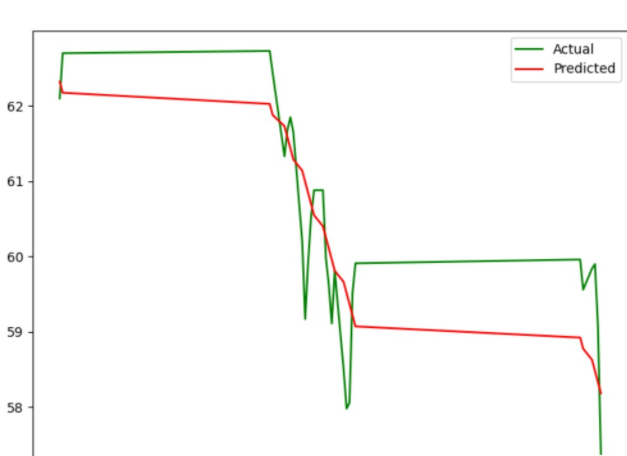可以看出预测的股票曲线虽然无法完全拟合真实股票走势，但是大体的趋势是一致的。另外值得一提的是，很多股票预测实验预测值与真实值贴的很紧密，这一般都是用今天的信息预测今天涨跌了，这种预测是无意义的。

4 最后-毕设帮助

毕设帮助，开题指导，技术解答
🇶746876041

展开全文HUXINY 2021-12-09 21:12:23
• HMM学习笔记2 隐马尔科夫模型与前向算法 机器学习 HMM 隐马尔科夫模型 前向算法 递归

bohu83 2020-11-26 14:42:49
• maverick17 2018-03-15 22:08:02...